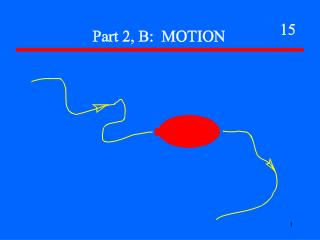Download PresentationFundamental Quantities and Units

# Fundamental Quantities and Units - PowerPoint PPT PresentationDownload Presentation## Fundamental Quantities and Units

- - - - - - - - - - - - - - - - - - - - - - - - - - - E N D - - - - - - - - - - - - - - - - - - - - - - - - - - -
##### Presentation Transcript

1. Fundamental Quantities and Units Fundamental Quantity MKS Unit * Length (Meters) * Mass (Kilograms) * Time (Seconds)

2. Motion

3. Motion The symbol “Δ” means “Change in”

4. Motion The symbol “Δ” means “Change in”

5. The speed changes by 4 m/sec every second

6. After 1 sec v = 7 m/sec

7. After 1 sec v = 7 m/sec After 2 sec v = 11 m/sec

8. After 1 sec v = 7 m/sec After 2 sec v = 11 m/sec After 3 sec v = 15 m/sec

9. Motion Relationships If a = 0 d = vt If a ≠ 0 d = ½ a t2

10. Motion An object is thrown vertically upward from the surface of the earth in the absence of air resistance. Note: this can be accomplished, for example, in an experimental facility from which the atmosphere has been removed. 1. What is the acceleration of the object on its way up? 2. What is the acceleration of the object on its way down? 3. If the object is thrown vertically, it will stop at the highest point in its motion. What is the acceleration of the object at the moment that it stops?

11. Motion Air Hockey There is no friction between the puck and the table surface

12. Motion Free Fall Tower d Drop distance - d 100 m y Height above base - y Assumption: No air resistance

13. Motion 1. Assume the air hockey puck moves with an initial speed of 10 m/s. What is its position (assume it starts a 0 m) after 1 s, 2 s, 3 s, 4 s and 5 s? 2. Assume the Free Fall Tower is 100 m high. What is the drop distance and the height above the base after 1 s, 2 s, 3 s, 4 s and 5 s? Assume g = 10 m/s2 .

14. Motion Can be a combination

15. Motion Can be a combination d = ½ a t2

16. Motion Can be a combination d = v t d = ½ a t2